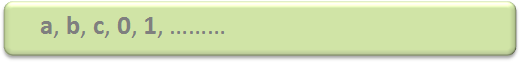# Introduction of Theory of Computation

• Difficulty Level : Basic
• Last Updated : 29 Oct, 2021

Automata theory (also known as Theory Of Computation) is a theoretical branch of Computer Science and Mathematics, which mainly deals with the logic of computation with respect to simple machines, referred to as automata.

Automata* enables scientists to understand how machines compute the functions and solve problems. The main motivation behind developing Automata Theory was to develop methods to describe and analyze the dynamic behavior of discrete systems.

Attention reader! Don’t stop learning now.  Practice GATE exam well before the actual exam with the subject-wise and overall quizzes available in GATE Test Series Course.

Learn all GATE CS concepts with Free Live Classes on our youtube channel.

Automata is originated from the word “Automaton” which is closely related to “Automation”.

Now, let’s understand the basic terminologies, which are important and frequently used in the Theory of Computation.

Symbol: Symbol(often also called character) is the smallest building block, which can be any alphabet, letter, or picture.Alphabets (Σ): Alphabets are a set of symbols, which are always finiteString: String is a finite sequence of symbols from some alphabet. A string is generally denoted as w and the length of a string is denoted as |w|

Note: Σ* is a set of all possible strings(often power set(need not be unique here or we can say multiset) of string) So this implies that language is a subset of Σ*.

```Empty string is the string with
zero occurrence of symbols,
represented as ε.```

```Number of Strings (of length 2)
that can be generated over the alphabet {a, b} -
-   -
a   a
a   b
b   a
b   b

Length of String |w| = 2
Number of Strings = 4

Conclusion:
For alphabet {a, b} with length n, number of
strings can be generated = 2n.```

Note – If the number of Σ’s is represented by |Σ|, then a number of strings of length n, possible over Σ is |Σ|n.

Language: A language is a set of strings, chosen from some Σ* or we can say- A language is a subset of Σ* . A language that can be formed over ‘ Σ ‘ can be Finite or Infinite.

```Example of Finite Language:
L1 = { set of string of 2 }
L1 = { xy, yx, xx, yy }

Example of Infinite Language:
L1 = { set of all strings starts with 'b' }
L1 = { babb, baa, ba, bbb, baab, ....... }```
My Personal Notes arrow_drop_up